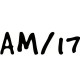# CBSE Class 12 Maths Notes Differential EquationsWritten by

Differential Equations is part of Class 12 Maths Notes for Quick Revision. Here we have given Class 12 Maths Notes Differential Equations.Differential Equations

Differential Equation: An equation involving independent variable, dependent variable, derivatives of dependent variable with respect to independent variable and constant is called a differential equation.
e.g.Ordinary Differential Equation: An equation involving derivatives of the dependent variable with respect to only one independent variable is called an ordinary differential equation.
e.g.From any given relationship between the dependent and independent variables, a differential equation can be formed by differentiating it with respect to the independent variable and eliminating arbitrary constants involved.

Order of a Differential Equation: Order of a differential equation is defined as the order of the highest order derivative of the dependent variable with respect to the independent variable involved in the given differential equation.
Note: Order of the differential equation, cannot be more than the number of arbitrary constants in the equation.

Degree of a Differential Equation: The highest exponent of the highest order derivative is called the degree of a differential equation provided exponent of each derivative and the unknown variable appearing in the differential equation is a non-negative integer.
Note
(i) Order and degree (if defined) of a differential equation are always positive integers.
(ii) The differential equation is a polynomial equation in derivatives.
(iii) If the given differential equation is not a polynomial equation in its derivatives, then its degree is not defined.

Formation of a Differential Equation: To form a differential equation from a given relation, we use the following steps:
Step I: Write the given equation and see the number of arbitrary constants it has.
Step II: Differentiate the given equation with respect to the dependent variable n times, where n is the number of arbitrary constants in the given equation.
Step III: Eliminate all arbitrary constants from the equations formed after differentiating in step (II) and the given equation.
Step IV: The equation obtained without the arbitrary constants is the required differential equation.

## Solution of the Differential Equation

A function of the form y = Φ(x) + C, which satisfies given differential equation, is called the solution of the differential equation.
General solution: The solution which contains as many arbitrary constants as the order of the differential equation, is called the general solution of the differential equation, i.e. if the solution of a differential equation of order n contains n arbitrary constants, then it is the general solution.

Particular solution: A solution obtained by giving particular values to arbitrary constants in the general solution of a differential equation, is called the particular solution.

## Methods of Solving First Order and First Degree Differential Equation

Variable separable form: Suppose a differential equation is$Differential Equations$ = F(x, y). Here, we separate the variables and then integrate both sides to get the general solution, i.e. above equation may be written as$\frac { dy }{ dx }$ = h(x) . k(y)
Then, by separating the variables, we get$Differential Equations$ = h(x) dx.
Now, integrate above equation and get the general solution as K(y) = H(x) + C
Here, K(y) and H(x) are the anti-derivatives of$\frac { 1 }{ K(y) }$ and h(x), respectively and C is the arbitrary constant.

Homogeneous differential equation: A differential equation$\frac { dy }{ dx } =\frac { f(x,y) }{ g(x,y) }$ is said to be homogeneous, if f(x, y) and g(x, y) are homogeneous functions of same degree, i.e. it may be written asTo check that given differential equation is homogeneous or not, we write differential equation as$\frac { dy }{ dx }$ = F(x, y) or$\frac { dx }{ dy }$ = F(x, y) and replace x by λx, y by λy to write F(x, y) = λ F(x, y).
Here, if power of λ is zero, then differential equation is homogeneous, otherwise not.

Solution of homogeneous differential equation: To solve homogeneous differential equation, we put
y = vx$\frac { dy }{ dx }$ = v + x$\frac { dv }{ dx }$
in Eq. (i) to reduce it into variable separable form. Then, solve it and lastly put v =$\frac { y }{ x }$ to get required solution.

Note: If the homogeneous differential equation is in the form of$\frac { dy }{ dx }$ = F(x, y), where F(x, y) is homogeneous function of degree zero, then we make substitution$\frac { x }{ y }$ = v, i.e. x = vy and we proceed further to find the general solution as mentioned above.

Linear differential equation: General form of linear differential equation is$\frac { dy }{ dx }$ + Py = Q …(i)
where, P and Q are functions of x or constants.
or$\frac { dx }{ dy }$ + P’x = Q’ …(ii)
where, P’ and Q’ are functions of y or constants.
Then, solution of Eq. (i) is given by the equation
y × IF = ∫(Q × IF) dx + C
where, IF = Integrating factor and IF = e∫Pdx
Also, solution of Eq. (ii) is given by the equation
x × IF = ∫ (Q’ × IF) dy + C
where, IF = Integrating factor and IF = e∫P’dy

We hope the given CBSE Class 12 Maths Notes Differential Equations will help you. If you have any query regarding NCERT Class 12 Maths Notes Differential Equations, drop a comment below and we will get back to you at the earliest.

## Class 12 Maths Notes

Relations and Functions

Inverse Trigonometric Functions

Matrices

Determinants

Continuity and Differentiability

Application of Derivatives

Integrals

Application of Integrals

Differential Equations

Vector Algebra

Three Dimensional Geometry

Linear Programming

Probability